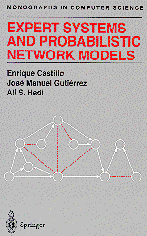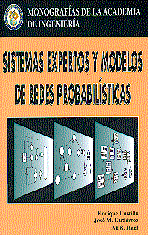Expert Systems and Probabilistic Network Models

(Springer-Verlag, 1997)

Sistemas Expertos y Modelos de Redes Probabilísticas

E. Castillo, J.M. Gutiérrez, and A.S. Hadi

# English Version  Spanish VersionSpringer-Verlag, New York (1997)Monografías de la Academia Española de Ingeniería, Madrid (1998) Buy it in Springer catalogue Buy it in Amazon.com Solicita información para adquirir una copia encuadernada del libro

(más información).

 Rule-based expert systems [English] [Spanish] Probability-based expert systems [English] [Spanish] Introduction to probabilistic networks [English] [Spanish] Gaussian probabilistic networks [English] [Spanish] Inference in probabilistic networks [English] [Spanish] Approximate inference (simulation) [English] [Spanish] Symbolic techniques [English] [Spanish]

Expert systems and uncertainty in artificial intelligence have seen a great surge of research activity during the last decade. This book provides a clear and up-to-date account of the research progress in these areas.

The authors begin with a survey of rule-based expert systems, which are mainly applicable to deterministic situations. Since most practical applications involve some degree of uncertainty, the authors then introduce probabilistic expert systems to deal with this element of uncertainty. They build on this foundation by showing how coherent expert systems are constructed and how probabilistic models such as Bayesian and Markov networks are developed. Subsequent chapters discuss how knowledge is updated by using both exact and approximate propagation methods. Other subjects such as symbolic propagation, sensitivity analysis, and learning are also presented. The book concludes with a chapter that applies the methods presented in the book to some case studies of real-life applications.

The concepts, ideas, and algorithms are illustrated by more than 150 examples and more than 250 graphs with the aid of computer programs developed by the authors. These programs can be obtained from a World Wide Web site (see the address in the preface). The book also includes end-of-chapter exercises and an extensive bibliography.

This book is intended for advanced undergraduate and graduate students, and for research workers and professionals from a variety of fields, including computer science, applied mathematics, statistics, engineering, medicine, business, economics, and social sciences. No previous knowledge of expert systems is assumed. Readers are assumed to have some background in probability and statistics.

1. Introduction

1.1 Introduction 1
1.2 What Is an Expert System? 2
1.3 Motivating Examples 3
1.4 Why Expert Systems? 7
1.5 Types of Expert System 8
1.6 Components of an Expert System 10
1.7 Developing an Expert System 14
1.8 Other Areas of AI 16
1.9 Concluding Remarks 20

2. Rule-Based Expert Systems

2.1 Introduction 21
2.2 The Knowledge Base 22
2.3 The Inference Engine 28
2.4 Coherence Control 48
2.5 Explaining Conclusions 52
2.6 Some Applications 53
2.7 Introducing Uncertainty 65
Exercises 65

3. Probabilistic Expert Systems

3.1 Introduction 69
3.2 Some Concepts in Probability Theory 71
3.3 Generalized Rules 85
3.4 Introducing Probabilistic Expert Systems 86
3.5 The Knowledge Base 91
3.6 The Inference Engine 102
3.7 Coherence Control 104
3.8 Comparing Rule-Based and Probabilistic Expert Systems 106
Exercises 108

4. Some Concepts of Graphs

4.1 Introduction 113
4.2 Basic Concepts and Definitions 114
4.3 Characteristics of Undirected Graphs 118
4.4 Characteristics of Directed Graphs 122
4.5 Triangulated Graphs 129
4.6 Cluster Graphs 139
4.7 Representation of Graphs 144
4.8 Some Useful Graph Algorithms 158
Exercises 172

5. Building Probabilistic Models

5.1 Introduction 175
5.2 Graph Separation 177
5.3 Some Properties of Conditional Independence 184
5.4 Special Types of Input Lists 192
5.5 Factorizations of the JPD 195
5.6 Constructing the JPD 200
Appendix to Chapter 5 204
Exercises 206

6. Graphically Specified Models

6.1 Introduction 211
6.2 Some Definitions and Questions 213
6.3 Undirected Graph Dependency Models 218
6.4 Directed Graph Dependency Models 237
6.5 Independence Equivalent Graphical Models 252
6.6 Expressiveness of Graphical Models 259
Exercises 262

7. Extending Graphically Specified Models

7.1 Introduction 267
7.2 Models Specified by Multiple Graphs 269
7.3 Models Specified by Input Lists 275
7.4 Multifactorized Probabilistic Models 279
7.5 Multifactorized Multinomial Models 279
7.6 Multifactorized Normal Models 292
7.7 Conditionally Specified Probabilistic Models 298
Exercises 311

8. Exact Propagation in Probabilistic Network Models

8.1 Introduction 317
8.2 Propagation of Evidence 318
8.3 Propagation in Polytrees 321
8.4 Propagation in Multiply-Connected Networks 342
8.5 Conditioning Method 342
8.6 Clustering Methods 351
8.7 Propagation Using Join Trees 366
8.8 Goal-Oriented Propagation 377
8.9 Exact Propagation in Gaussian Networks 382
Exercises 387

9. Approximate Propagation Methods

9.1 Introduction 393
9.2 Intuitive Basis of Simulation Methods 394
9.3 General Frame for Simulation Methods 400
9.4 Acceptance-Rejection Sampling Method 406
9.5 Uniform Sampling Method 409
9.6 The Likelihood Weighing Sampling Method 411
9.7 Backward-Forward Sampling Method 413
9.8 Markov Sampling Method 415
9.9 Systematic Sampling Method 419
9.10 Maximum Probability Search Method 429
9.11 Complexity Analysis 439
Exercises 440

10. Symbolic Propagation of Evidence

10.1 Introduction 443
10.2 Notation and Basic Framework 445
10.3 Automatic Generation of Symbolic Code 447
10.4 Algebraic Structure of Probabilities 454
10.5 Symbolic Propagation Through Numeric Computations 455
10.6 Goal-Oriented Symbolic Propagation 464
10.7 Symbolic Treatment of Random Evidence 470
10.8 Sensitivity Analysis 472
10.9 Symbolic Propagation in Gaussian Bayesian Networks 474
Exercises 478

11. Learning Bayesian Networks

11.1 Introduction 481
11.2 Measuring the Quality of a Bayesian Network Model 484
11.3 Bayesian Quality Measures 486
11.4 Bayesian Measures for Multinomial Networks 490
11.5 Bayesian Measures for Multinormal Networks 499
11.6 Minimum Description Length Measures 506
11.7 Information Measures 509
11.8 Further Analyses of Quality Measures 509
11.9 Bayesian Network Search Algorithms 511
11.10 The Case of Incomplete Data 513
Appendix to Chapter 11: Bayesian Statistics 515
Exercises 525

12. Case Studies

12.1 Introduction 529
12.2 Pressure Tank System 530
12.3 Power Distribution System 542
12.4 Damage of Concrete Structures 550
12.5 Damage of Concrete Structures: The Gaussian Model 562
Exercises 567

List of Notation
References
Index